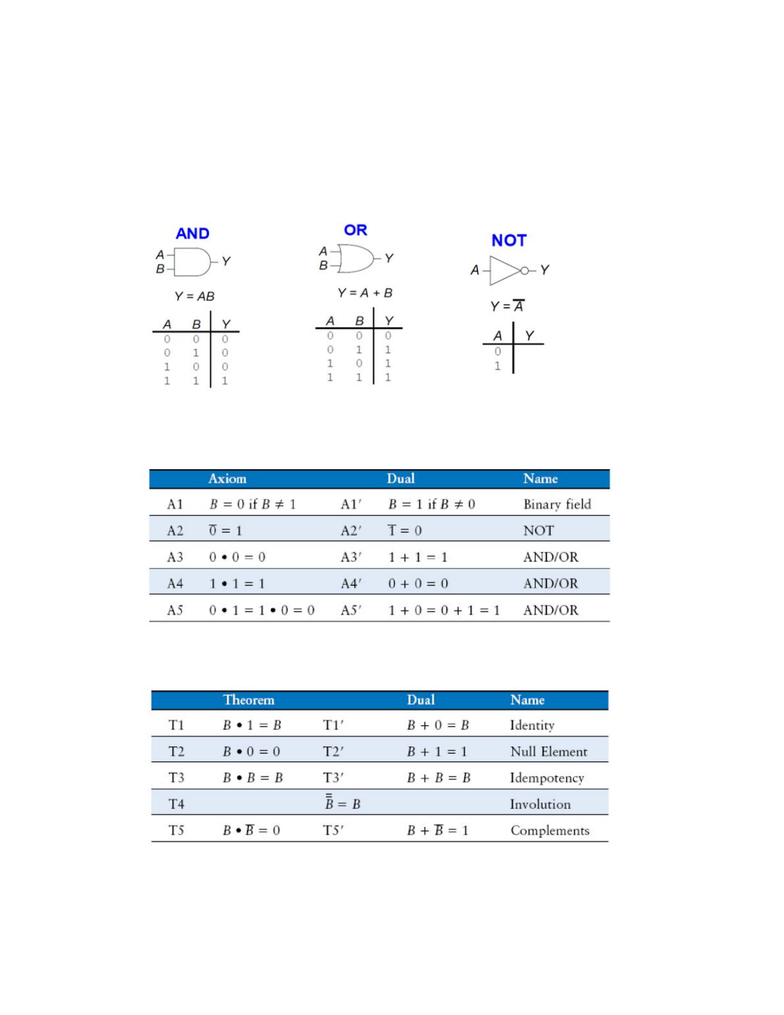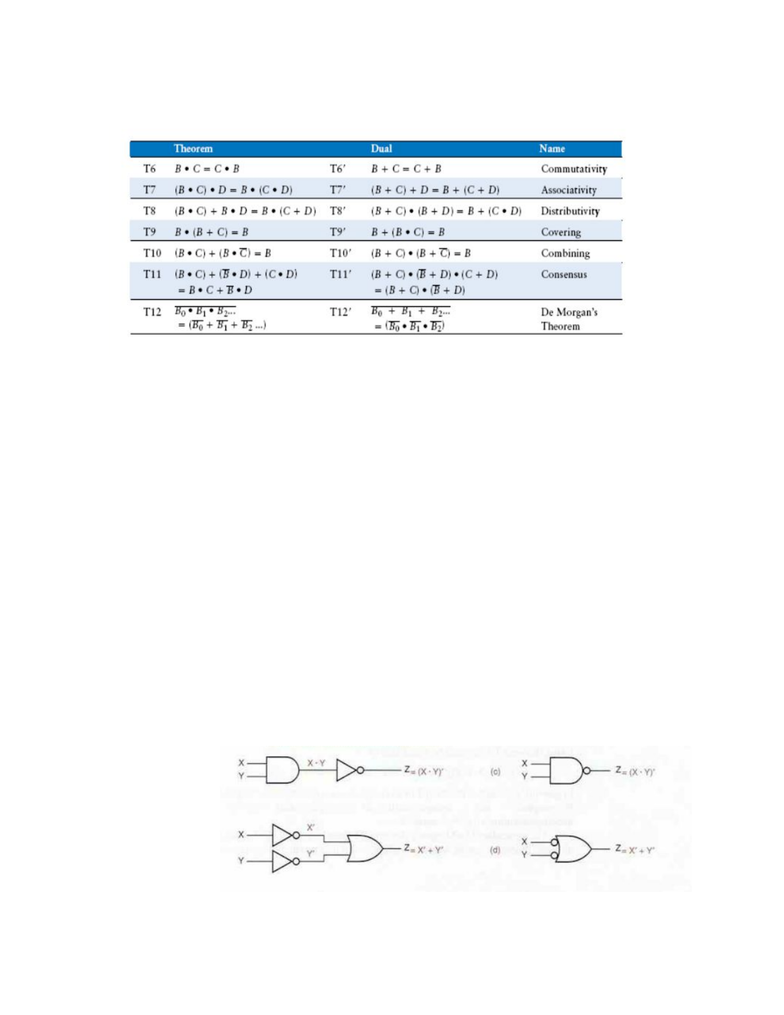# 14:332:231 Lecture Notes - Lecture 3: Logic Gate, Summation, Logic Optimization

62 views6 pagesCHAPTER FOUR: COMBINATIONAL LOGIC DESIGN PRINCIPLES
4.1 Switching Algebra
Use positive-logic convention, where 0 is LOW and 1 is HIGH
3 Basic Logic Gates
Axioms
Theorems
Unlock document

This preview shows pages 1-2 of the document.
Unlock all 6 pages and 3 million more documents.Theorems of Several Variables
Precedence: multiplication takes precedence over addition
Theorems Explained
Covering Theorem (T9)
We only need to include X in the expression
X is said to cover
X*Y
Combining Theorem (T10)
If the subexpression X*Y + X*Y’ appears in an expression, we can then
replace it with just X
Consensus Theorem (T11)
The Y*Z term is called the consensus
of X*Y and X’*Z
If Y*Z is 1, then either X*Y or X’*Z must also be one
Therefore, term Y*Z is redundant and can be dropped
Theorems of Many Variables Explained
Most of these theorems can be proved using a two-step method called finite
induction
First proving the theorem is true for n=2
Then proving the theorem is true for n=i and n=i+1 (induction step
)
DeMorgan’s Theorem
Examples
Unlock document

This preview shows pages 1-2 of the document.
Unlock all 6 pages and 3 million more documents.